# 铁路货运通道上的单组列车编组计划优化算法研究Research on Optimization Algorithms of Single-Block Train Formation Plan on Railway Corridor

DOI: 10.12677/AAM.2020.91003, PDF, HTML, XML, 下载: 229  浏览: 350  科研立项经费支持

Abstract: This paper studies the optimization problem of single-block train formation plan on railway corridor. Railway corridor is the backbone of the railway network. When applying the existing research results on train formation plan of general railway network to railway corridor, due to the characteristics of railway corridor itself and traffic flow are not fully considered in modeling, the computational resources consumption is large and the optimization effect is general. In order to solve the above problems, this paper establishes an optimization model of single-block train formation plan on the railway corridor with the time cost as the main optimization objective, and designs two iterative algorithms based on greedy strategy for the model. Numerical experiments show that, compared with the common genetic algorithm, the method adopted in this paper is faster and saves the time cost by more than 14% on average.

1. 引言

1.1. 背景介绍

1.2. 相关工作

1.3. 本文贡献

1) 通过对货运通道本身特点以及货运通道上车流特征的分析，指出对于货运通道上的列车编组计划优化问题，经济成本优化与时间成本优化具有一致性，并由此建立了以列车集结时间以及货物中转时间为主要优化目标的单组列车编组计划优化模型。

2) 针对上述货运通道上的单组列车编组计划优化模型，设计了两种基于贪婪策略的迭代算法，并且通过数值实验说明其拥有更好的优化结果与更快的运算速度。

2. 模型

2.1. 模型描述

2.2. 模型公式

${N}_{ij}$ ：从i站到j站的车流路径所包含的技术站的集合，不包含始发站和终到站；

${g}_{ij}$ ：从i站到j站的编组去向吸收的车流量；：需求s的原始车流量大小；

${m}_{ij}$ ：从i站到j站的列车的平均编成辆数，本文取该值为55；

${c}_{i}$ ：技术站i的列车集结时间；

${e}_{k}$ ：沿途中间技术站k节省的时间。

${x}_{ij}$ ：当建立从i站到j站的编组去向时，其值为1，否则为0；

${y}_{ij}^{s}$ ：当需求s在运输途中编入从i站到j站的编组去向时，其值为1，否则为0；

$\underset{j\in V}{\sum }{y}_{ij}^{s}=1,\text{\hspace{0.17em}}\text{\hspace{0.17em}}\forall i\in V,\text{\hspace{0.17em}}\forall s\in S$

$\underset{j\in V}{\sum }{y}_{o\left(s\right)j}^{s}=1,\text{\hspace{0.17em}}\text{\hspace{0.17em}}o\left(s\right)为需求s的起点$

$\underset{j\in V}{\sum }{y}_{jd\left(s\right)}^{s}=1,\text{\hspace{0.17em}}\text{\hspace{0.17em}}d\left(s\right)为需求s的终点$

$\underset{i\in V}{\sum }{y}_{ik}^{s}-\underset{j\in V}{\sum }{y}_{kj}^{s}=0,\text{\hspace{0.17em}}\text{\hspace{0.17em}}k为需求s的中间点$

${g}_{ij}=\underset{s\in S}{\sum }{y}_{ij}^{s}\cdot {p}_{s},\text{\hspace{0.17em}}\text{\hspace{0.17em}}\forall i\in V,j\in V$

${t}_{ij}=\underset{k\in {N}_{ij}}{\sum }{e}_{k},\text{\hspace{0.17em}}\text{\hspace{0.17em}}\forall i\in V,j\in V$

$Z=\mathrm{max}\underset{i,j\in V}{\sum }{t}_{ij}\cdot {g}_{ij}-\underset{i,j\in V}{\sum }{c}_{i}{x}_{ij}{m}_{ij}$

3. 算法设计

3.1. 贪婪算法一

3.2. 贪婪算法二

3.3. 遗传算法

4. 数值实验

4.1. 参数设置

4.2. 实验结果

Table 1. Comparison of experimental results

5. 结论与展望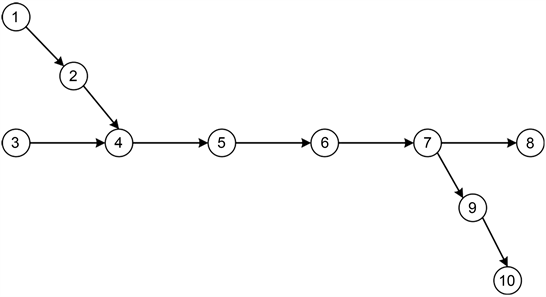Figure A1. Road network diagram of freight transportation corridorTable A1. Parameters of each technical stationTable A2. Traffic flow path for each demand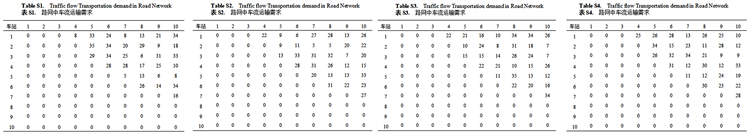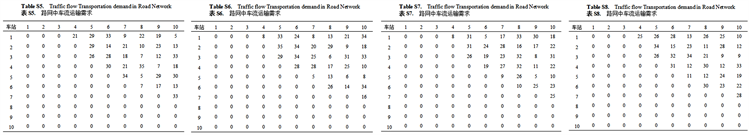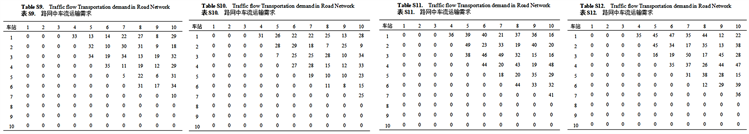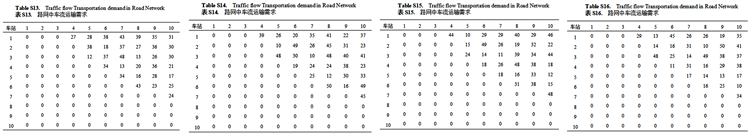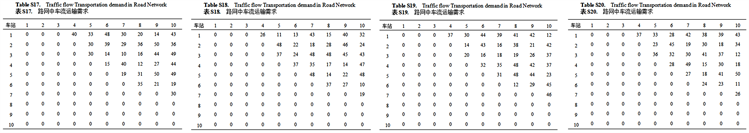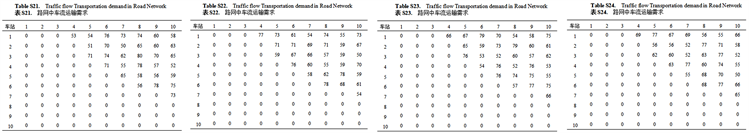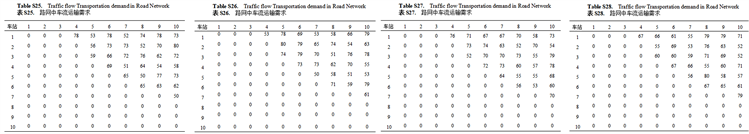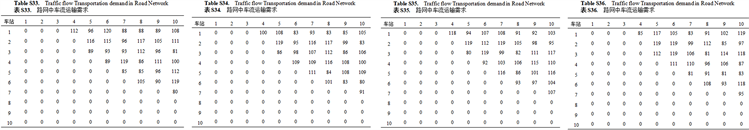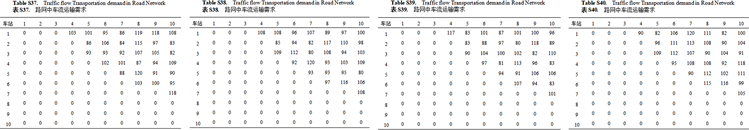Table A3. Traffic flow transportation demand in road network

NOTES

*通讯作者。

  陈崇双, 王慈光, 薛锋, 等. 货物列车编组计划国内外研究综述[J]. 铁道学报, 2012, 34(2): 8-20.  胡思继. 铁路行车组织[M]. 第2版. 北京: 中国铁道出版社, 2014: 104-105.  严贺祥. 铁路货运通道布局优化的模型和方法研究[D]: [博士学位论文]. 北京: 北京交通大学, 2008.  余巧凤, 梁栋. 铁路运输通道现状分析与发展设想[J]. 铁道经济研究, 2009(2): 27-30 + 45.  Li, Y.H., Wu, S.G. and Peng, Q.Y. (2002) Network Model and Algorithm for Freight Train Marshalling Plan. Journal of Southwest Jiaotong University, 37, 68-71.  Angel, M. and Javier, S. (2007) Tactical Design of Rail Freight Networks. II: Local Search Methods with Statistical Analysis. European Journal of Operational Research, 94, 43-53. https://doi.org/10.1016/0377-2217(95)00193-X  Ahuja, R.K., Jha, K.C. and Liu, J. (2007) Solving Real-Life Railroad Blocking Problems. Interfaces, 37, 404-419. https://doi.org/10.1287/inte.1070.0295  林柏梁, 朱松年. 优化编组计划的非线性0-1规划模型及模拟退火算法[J]. 铁道学报, 1994(2): 61-66.  杨时刚, 史峰, 李致中. 制定列车编组计划的人工神经网络方法[J]. 铁道科学与工程学报, 2002, 20(3): 79-84.  Yaghini, M., Foroughi, A. and Nadjari, B. (2011) Solving Railroad Blocking Problem Using Ant Colony Optimization Algorithm. Applied Mathematical Modelling, 35, 5579-5591. https://doi.org/10.1016/j.apm.2011.05.018  许红, 马建军, 龙昭, 等. 技术站单组列车编组方案模型与计算方法的研究[J]. 铁道学报, 2006, 28(3): 12-17.  左武. 铁路分组货物列车编组计划的遗传算法研究[D]: [博士学位论文]. 长沙: 中南大学, 2009.  杜祜康, 赵英凯. 整数规划问题智能求解算法综述[J]. 计算机应用研究, 2010, 27(2): 408-412.  王保华, 何世伟. 铁路车流改编方案随机优化模型及其算法[J]. 中国铁道科学, 2009, 30(5): 104-108.  马宏朋. 实用型路网列车编组计划优化模型与算法的研究与实现[D]: [博士学位论文]. 北京: 北京交通大学, 2017.  Barnhart, C., Jin, H. and Vance, P.H. (2000) Railroad Blocking: A Network Design Application. Operations Research, 48, 603-614. https://doi.org/10.1287/opre.48.4.603.12416